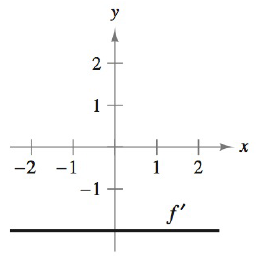Chapter 5.1, Problem 40E### Calculus: An Applied Approach (Min...

10th Edition
Ron Larson
ISBN: 9781305860919

#### Solutions

Chapter
Section### Calculus: An Applied Approach (Min...

10th Edition
Ron Larson
ISBN: 9781305860919
Textbook Problem
1 views

# Interpreting a Graph In Exercises 39–42, the graph of the derivative of a function is given. Sketch the graphs of two functions that have the given derivative. (There is more than one correct answer.)To determine

To graph: The two functions of the derivative whose graph is shown by,Explanation

Given Information:

The graph of the provided derivative is shown below.

Graph:

From the graph, the derivative of the function is given as,

f(x)=2

Integrate the above derivative function use the basic rule of integration kdx=kx+C.

f(x)dx=2dxf(x)=2x+C

The value of C is any constant. It can by any numerical value.

Substitute 0 for C in above function.

f(x)=2x+0

Substitute 1 for C in above function.

f(x)=2x+1

Therefore, the function whose derivative is (f(x)=2) are f(x)=2x or f(x)=2x+1.

Graph the function f(x)=2x. It is linear function so the graph of this function is straight line.

Now, choose some values for x and compute the value for f(x), in order to form the ordered pairs that will plot on the graph.

So, put x=1 in the function f(x)=2x.

f(1)=2(1)=2

Now, put x=0 in the function f(x)=2x.

f(0)=2(0)=0

Now, put x=1 in the function f(x)=2x.

f(1)=2(1)=2

The required table is shown below,

 x f(x) (x,f(x)) −1 2 (−1,2) 0 0 (0,0) 1 −2 (1,−2)

Therefore, the graph of the function f(x)=2x as shown below,

### Still sussing out bartleby?

Check out a sample textbook solution.

See a sample solution

#### The Solution to Your Study Problems

Bartleby provides explanations to thousands of textbook problems written by our experts, many with advanced degrees!

Get Started

#### Multiply:

Mathematical Applications for the Management, Life, and Social Sciences

#### Evaluate the indefinite integral. sec22d

Single Variable Calculus: Early Transcendentals

#### True or False: If f(x) 0 for all x in an interval I then f is increasing on I.

Study Guide for Stewart's Single Variable Calculus: Early Transcendentals, 8th